# Triangular Elements. Barycentric Coordinates.

## Barycentric Coordinates

For each point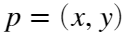, in this triangle one can define the barycentric coordinades of this point using the value of the 2D shape functions defined for this triangle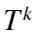: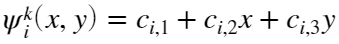satisfying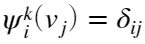.
This way, one can express any point using three coordinates (barycentric)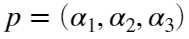. In particular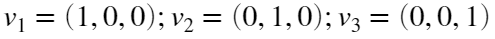.
The barycentric coordinates satisfy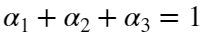. Moreover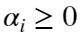unless the point is outside of the triangle.
(see https://en.wikipedia.org/wiki/Barycentric_coordinate_system )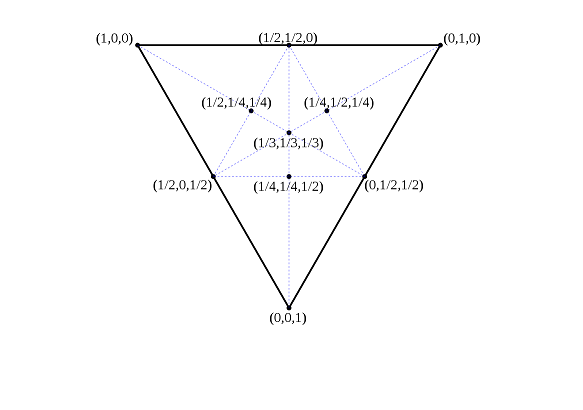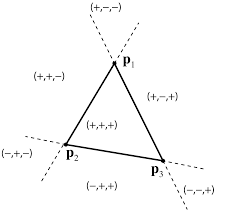## Compute Barycentric Coordinates

Given a triangle defined by vertices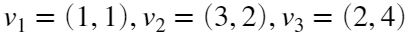, compute the barycentric coordinates of point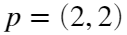.
Verify that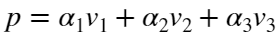.
p=[2,2]; %point
vertices=[1,1; %define the triangle vertex coordinates
3,2;
2,4];
vplot=[vertices;vertices(1,:)]; %just to plot a closed triangle, we add the first point at the end
plot(vplot(:,1),vplot(:,2));
hold on
plot(p(1,1),p(1,2),'o');
hold off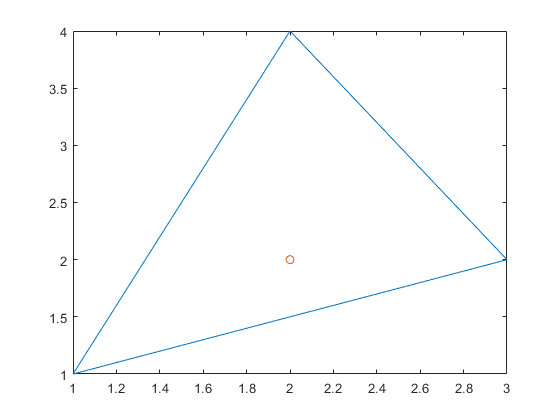Compute shape functions
A=[ones(3,1), vertices];
b=[1;0;0];
c1=A\b;
b=[0;1;0];
c2=A\b;
b=[0;0;1];
c3=A\b;
Psi1 = @(x,y) c1(1)+c1(2)*x+c1(3)*y;
Psi2 = @(x,y) c2(1)+c2(2)*x+c2(3)*y;
Psi3 = @(x,y) c3(1)+c3(2)*x+c3(3)*y;
%
% Compute barycentric coordinates
%
alpha1 = Psi1(p(1),p(2));
alpha2 = Psi2(p(1),p(2));
alpha3 = Psi3(p(1),p(2));
[alpha1,alpha2,alpha3] %results
ans = 1×3
0.4000 0.4000 0.2000
alpha1+alpha2+alpha3-1 %check error
ans = 0
%
% Verification
%
p-(alpha1*vertices(1,:)+alpha2*vertices(2,:)+alpha3*vertices(3,:))
ans = 1×2
0 0

## Exercise 1: Build baryCoord function

Build a function file that returns the barycentric coordinates of a point with respect to a triangle. It must return a flag value isInside with value 1 if the point is inside the triangle and 0 if it is outside.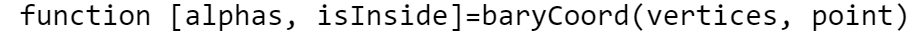Use the previous example to check if it works.

## Exemple: Correspondence with the Reference Triangle

Usually the reference triangular element,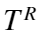, is the triangle defined by the vertices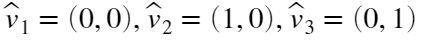We can use barycentric coordinates to make a correspondence between the reference triangle and every other triangle. Let's consider the preivous exemple triangleAssuming that each vertex has its correspondence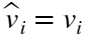, using the barycentric coordinates we obtain the point correspondences: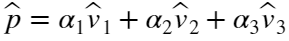.
verticesTR=[0,0; %define the reference triangle (TR) vertex coordinates
1,0;
0,1];
plot(vplot(:,1),vplot(:,2));
hold on
axis equal;
plot(p(1,1),p(1,2),'o');
%compute the corresponding point on the reference triangle
pTR= alpha1*verticesTR(1,:)+alpha2*verticesTR(2,:)+alpha3*verticesTR(3,:)
pTR = 1×2
0.4000 0.2000
vplotTR=[verticesTR;verticesTR(1,:)]; %just to plot a closed triangle, we add the first point at the end
plot(vplotTR(:,1),vplotTR(:,2));
plot(pTR(1,1),pTR(1,2),'s');
linePlot=[p; pTR]
linePlot = 2×2
2.0000 2.0000 0.4000 0.2000
plot(linePlot(:,1),linePlot(:,2),'.-');
hold off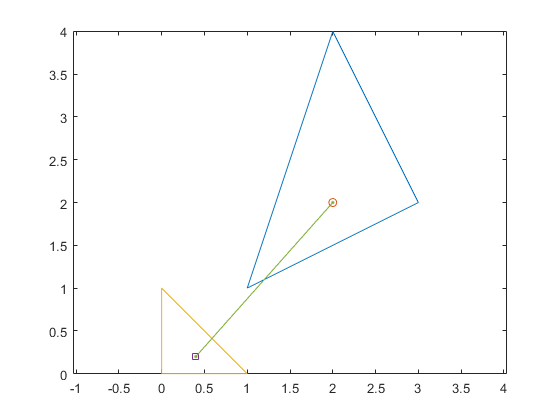## Exercise 1.1: Point correspondence

Consider now the obtained point on the Reference Triangle pTR=(0.4,0.2) and compute the associated point on the Original Triangle. Observe that the barycentric coordinates are the same and the point obtained is (2,2)

## Exercise 2: Several Triangles

Let's consider now four triangles adding a central vertex (as it is shown in the figure). We can define these polygons by the coordinates of the vertices and the triangles defined by these vertices.
vertex=[
0,0;
1,0;
1,1;
0,1;
0.5,0.5;
];
triang=[
1, 2, 5;
2, 3, 5;
3, 4, 5;
4, 1, 5;
];
figure()
plotElements(vertex,triang,1); %find this file at *Numerical Factory*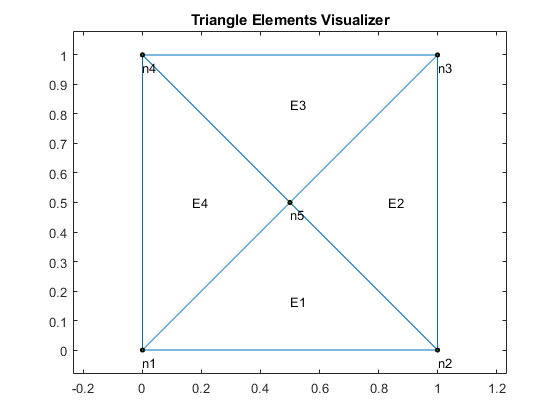## Exercise 2.1: Several Triangles

Use the function build in the previous exerciseto decide if the point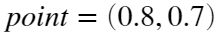belongs to each triangle
Solution= It belongs to triangle 2.

## ShapeFunctions2D Pract: Interpolate Temperature in a Triangle Mesh

A triangle mesh is defined by a set of nodes (the vertices coordinates) and a set of elements (the triangles) defined by the indices corresponding to the vertices belonging to each triangle (connectivity matrix).
The usual format is
nodes=[ x1,y1; x2,y2; ......; xN,yN]; a Nx2 matrix for N vertices
elements =[ i1,j1,k1; i2,j2,k2; .....; iM,jM,kM]; a Mx3 matrix for M triangles
Compute the temperature at the point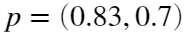Get the meshFiles file from Numerical Factory
eval('meshHole'); %load data from the file mesHole.m: nodes and elements
figure()
plotElements(vertex,triang,0);
hold on;
p=[0.83,0.7];
plot(p(1),p(2),'o')
hold off;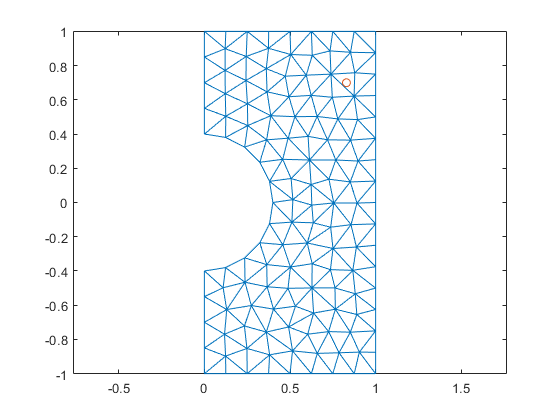assing a simple temperature value for each vertex. For now Temp=numNode this way the first nodes are cooler than the last ones.
Temp=1:size(nodes,1);

## Final step: Internal point interpolation

Finally find the triangle where the point p belongs to (using the baryCoord function) and interpolate the temperature value.
The idea of the code can be:
for all the elements
compute the barycentric coordinates
if (isInside >= 1), pick the element number; break; end;
end
Take this element and perform the interpolation using the temperatures of the nodes and barycentric coord (see theory presentation).
Solution: Temp= 104.0420; element= 189; nodes=[109, 112, 92];
(c)Numerical Factory 2020